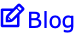Learning Pages

## Catch Ball

Home | Icebreakers | Introductions | Catch Ball

 This is a good exercise to use for introductions and for reinforcing concepts. Instead of going round the class in order when making introductions etc, throw a ball (preferably a soft one) at one of the students who then does the first introduction. This student then throws the ball to someone else. Challenge the class to complete the introductions without throwing the ball to the same person twice. Trainers often use questions to reinforce concepts. Instead of using 'pose then pounce' or 'pounce then pose' techniques, try asking the question as you throw a ball to the student ('throw and pose' technique). Throwing the ball is not a gimmick: the idea is to distract the student's conscious attention so that the answer can surface from the subconscious. Suitable balls for this exercise are either sponge balls or balls that have been constructed from strands of rubber in the fashion of a pompom. It's probably a good idea to clear the coffee cups before starting these exercises. Suitable balls for this exercise are either sponge balls or balls that have been constructed from strands of rubber in the fashion of a pompom.

Introductions